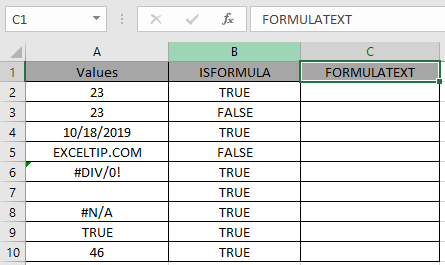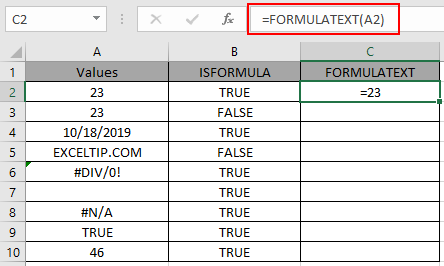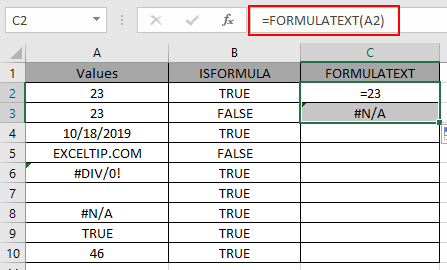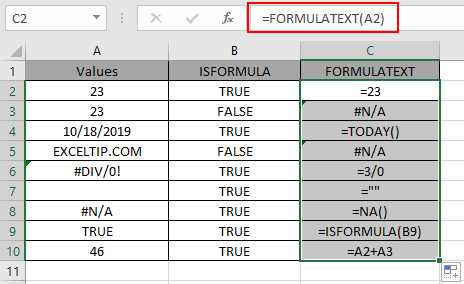# How to use the FORMULATEXT Function in Excel

FORMULA values in cell is the value returned by the formula. In Excel when there is a Formula in the cell, it starts with equals ( = ) sign. FORMULATEXT function returns TRUE if the cell has a formula which returns output or else False. Sometimes an empty cell is output of a function which returns an empty string.

ISFORMULA function is generally used to check that any formula is there in the cell or not.

FORMULATEXT function returns the formula of the reference cell if exist and returns the #NA! error if not.

Syntax:

 = FORMULATEXT ( reference )

reference : reference to a cell or range of cells.

Example:

Now let’s understand the function more via using it in an example.Here we have a list of output value in Values column and in ISFORMULA column we have results whether the reference cell has a formula or not.

ISFORMULA function is generally used to view whether the reference cell has a formula or not.

We will extract the formula from the reference cell to the output.

Use the formula:

 = FORMULATEXT ( A2 )

A2 : reference cell is provided to the function to check its value.As you can see the output 23 is a result of the formula =23 which returns a number 23.You can observe from the above snapshots that there is a difference between direct input value and return output value. In A2 cell there is  number which is returned by a formula ( = 23 ) whereas in A3 cell, the number is the value of the cell.

Copy the formula to other cells using the Ctrl + D shortcut key.As you can see, the function returns the formula of the reference cell or error if formula doesn’t exist.Below are the some of the observational notes.

Note:

1. Empty cell can have a formula in the cell. The formula returns an empty string by the formula.
2. When range of cells given as argument, the function only returns the result for the first cell of the array.
3. The function returns error if the reference to the function is invalid.
4. The reference cell can be of external open worksheet.
5. The function returns the #NA error if
1. the referenced cell doesn’t contain a formula.
2. the formula in the cell has more than 8192 characters.
3. If the worksheet protection doesn’t allow to view the formula.
4. If the external worksheet is not opened.
6. The function returns the #VALUE! Error if the invalid data types is used as input

You can use the ISFORMULA function to check the input reference of the cell.

Use the formula:

 = ISFORMULA ( reference )

Or you can view the formulas in the current worksheet using the keyboard shortcut

For windows : Ctrl + `

For Mac user : ^ + `

Hope you understood how to use FORMULATEXT function in Excel 2016. Find more articles on Excel ISLOGICAL function here. Please share your query below in the comment box. We will assist you.

Terms and Conditions of use

The applications/code on this site are distributed as is and without warranties or liability. In no event shall the owner of the copyrights, or the authors of the applications/code be liable for any loss of profit, any problems or any damage resulting from the use or evaluation of the applications/code.